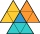# The Law of Cosines + sine - math problems

#### Number of problems found: 14

• Angles by cosine lawCalculate the size of the angles of the triangle ABC, if it is given by: a = 3 cm; b = 5 cm; c = 7 cm (use the sine and cosine theorem).
• ABCDAC= 40cm , angle DAB=38 , angle DCB=58 , angle DBC=90 , DB is perpendicular on AC , find BD and AD
• Largest angle of the triangleCalculate the largest angle of the triangle whose sides have the sizes: 2a, 3/2a, 3a
• Medians of isosceles triangleThe isosceles triangle has a base ABC |AB| = 16 cm and 10 cm long arm. What is the length of medians?
• Three vectorsThe three forces whose amplitudes are in ratio 9:10:17 act in the plane at one point to balance. Determine the angles of each two forces.
• Two chordsFrom the point on the circle with a diameter of 8 cm, two identical chords are led, which form an angle of 60°. Calculate the length of these chords.
• Triangle and its heightsCalculate the length of the sides of the triangle ABC, if va=5 cm, vb=7 cm and side b is 5 cm shorter than side a.
• Children playgroundThe playground has a trapezoid shape, and the parallel sides have a length of 36 m and 21 m. The remaining two sides are 14 m long and 16 m long. Find the size of the inner trapezoid angles.
• Viewing angleThe observer sees a straight fence 60 m long at a viewing angle of 30°. It is 102 m away from one end of the enclosure. How far is the observer from the other end of the enclosure?
• Triangle's centroidIn the triangle ABC the given lengths of its medians tc = 9, ta = 6. Let T be the intersection of the medians (triangle's centroid) and point is S the center of the side BC. The magnitude of the CTS angle is 60°. Calculate the length of the BC side to 2 d
• Two boatsTwo boats are located from a height of 150m above the surface of the lake at depth angles of 57° and 39°. Find the distance of both boats if the sighting device and both ships are in a plane perpendicular to the surface of the lake.
• Triangle SASCalculate the triangle area and perimeter, if the two sides are 51 cm and 110 cm long and angle them clamped is 130 °.
• Inner anglesThe inner angles of the triangle are 30°, 45° and 105° and its longest side is 10 cm. Calculate the length of the shortest side, write the result in cm up to two decimal places.
• Quadrilateral oblique prismWhat is the volume of a quadrilateral oblique prism with base edges of length a = 1m, b = 1.1m, c = 1.2m, d = 0.7m, if a side edge of length h = 3.9m has a deviation from the base of 20° 35' and the edges a, b form an angle of 50.5°.

We apologize, but in this category are not a lot of examples.
Do you have an interesting mathematical word problem that you can't solve it? Submit a math problem, and we can try to solve it.

We will send a solution to your e-mail address. Solved examples are also published here. Please enter the e-mail correctly and check whether you don't have a full mailbox.

Please do not submit problems from current active competitions such as Mathematical Olympiad, correspondence seminars etc...
Cosine rule uses trigonometric SAS triangle calculator. Most natural application of trigonometry and trigonometric functions is a calculation of the triangles. Common and less common calculations of different types of triangles offers our triangle calculator. Word trigonometry comes from Greek and literally means triangle calculation. The Law of Cosines - math problems. Sine - math problems.